#### Classical controllability

Let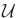denote the set of permissible action trajectories for the system, as considered in Section 14.1.1. By default, this is taken as any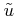for which (14.1) can be integrated. A system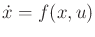is called controllable if for all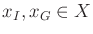, there exists a time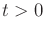and action trajectory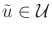such that upon integration from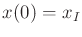, the result is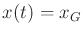. Controllability can alternatively be expressed in terms of the reachable sets of Section 14.2.1. The system is controllable if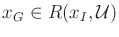for all.

A system is therefore controllable if a solution exists to any motion planning problem in the absence of obstacles. In other words, a solution always exists to the two-point boundary value problem (BVP).

Example 15..2 (Classical Controllability)   All of the vehicle models in Section 13.1.2 are controllable. For example, in an infinitely large plane, the Dubins car can be driven between any two configurations. Note, however, that if the plane is restricted by obstacles, then this is not necessarily possible with the Dubins car. As an example of a system that is not controllable, let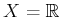,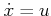, and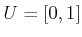. In this case, the state cannot decrease. For example, there exists no action trajectory that brings the state from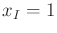to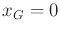.Many methods for determining controllability of a system are covered in standard textbooks on control theory. If the system is linear, as given by (13.37) with dimensions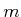and, then it is controllable if and only if the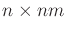controllability matrix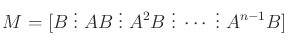(15.4)

has full rank . This is called the Kalman rank condition . If the system is nonlinear, then the controllability matrix can be evaluated on a linearized version of the system. Having full rank is sufficient to establish controllability from a single point (see Proposition 11.2 in ). If the rank is not full, however, the system may still be controllable. A fascinating property of some nonlinear systems is that they may be able to produce motions in directions that do not seem to be allowed at first. For example, the simple car given in Section 13.1.2 cannot slide sideways; however, it is possible to wiggle the car sideways by performing parallel-parking maneuvers. A method for determining the controllability of such systems is covered in Section 15.4.

For fully actuated systems of the form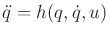, controllability can be determined by converting the system into double-integrator form, as considered in Section 14.4.1. Let the system be expressed as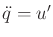, in which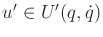. If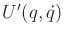contains an open neighborhood of the origin of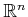, and the same neighborhood can be used for any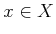, then the system is controllable. If a nonlinear system is underactuated, as in the simple car, then controllability issues become considerably more complicated. The next concept is suitable for such systems.

Steven M LaValle 2020-08-14